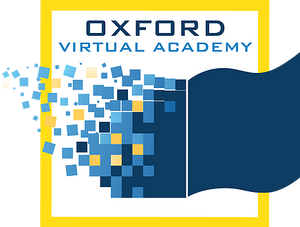# Trigonometry

This course addresses analyzing functions, transformations, and inverse functions. Students will also learn about radians, the unit circle, right-triangle trigonometry, trigonometric functions, inverse trigonometric functions, trigonometric identities, and trigonometric equations. Additional topics include vectors, conic sections, parametric curves, and the polar coordinate system.

* Prerequisites: Algebra II